# Tony’s Take April 2023

## This month’s topics:

### AI and mathematics.

Davide Castelvecchi, a staff writer for Nature, has covered developments in the interaction between artificial intelligence and mathematics over the last few years. As he explains it in his most recent posting (“How will AI change mathematics? Rise of chatbots highlights discussion”, in the issue dated March 2, 2023), this interaction has two very different modalities. Traditionally, humans relied on computers’ speed, accuracy and large memory for tedious computational tasks. An early example was the computer-assisted proof of the four-color theorem in 1976. In this line came proof assistants like Coq, first released in 1989. In fact, one of Coq’s first big jobs was checking that the four-color theorem proof actually worked. Proof assistants were also in the news more recently, in connection with Peter Scholze’s “Grand unification theory,” as reported by Castelvecchi in June 2021.

The other way AI has become involved in mathematics is through machine learning. Machine learning enabled computers to beat humans at chess and Go. Here, instead of being a super-secretary, the computer gets to learn on its own, through trial and error. This newer manifestation of artificial intelligence has recently become a topic of great public interest because of its packaging as “chatbots,” programs that have learned to imitate human speech patterns (specifically by training on sentence completion) to the point that they give the appearance of having learned to think. This has also been applied to mathematics. As Castelvecchi tells us, a group at Google has come up with a chatbot called Minerva, which has trained on a special corpus: mathematics arXiv postings. We learn that Minerva can accurately factor integers into primes in a certain range, but that once the numbers are too large it makes mistakes, “showing that it has not `understood’ the general procedure.” This is always going to be the problem with a program based on language.

Will computers ever be able to “do” math? Castelvecchi spoke with Melanie Mitchell (Santa Fe Institute), who says that our jobs are safe until AI can learn “to extract abstract concepts from concrete information.” That is, until computer programs can understand mathematical facts, and not just process mathematical words.

### Mouse bone-building cells prefer negative curvature.

A Nature Communications article published March 3, 2023 considers what happens when you grow cells on surfaces with varying principal curvatures.

What are principal curvatures? Consider a point P on a smooth surface in 3-dimensional space. Draw a vector ${\bf n}$ pointing outward, perpendicular to the surface at $P$. Now cut through the surface with a plane containing ${\bf n}$. The plane and the surface intersect along a curve; see some examples below.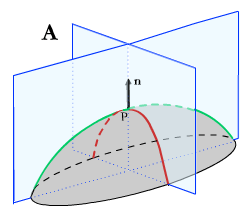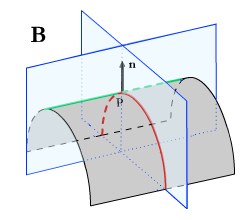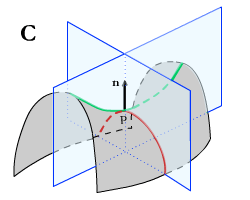In each case the curve with the maximum curvature at $P$ is shown in red, the one with minimum in green. In ${\bf A}$, both $\kappa$ are positive: the surface near $P$ is a convex dome. If both were negative, the dome would be concave. ${\bf B.}$ Here we see the principal curvature combination (positive, zero): near $P$ the surface is like a convex cylinder. (zero, negative) would make that cylinder concave and (zero, zero) would make the surface flat near $P$. ${\bf C.}$ The surface is saddle-shaped near $P$ .

For each of these curves, measure the curvature at $P$ by calculating 1 over the radius of the circle that best approximates the curve at $P$. Count it as positive if it curves away from ${\bf n}$. Generally at $P$ there will be a maximum $\kappa_1$ and a minimum $\kappa_2$ of these numbers. These are the principal curvatures at $P$.

The authors, a team of biomechanical engineers based mostly at the Delft University of Technology and led by Sebastien J. P. Callens and Amir Zadpoor, propose “a geometric perspective on cell-environment interactions that could be harnessed in tissue engineering and regenerative medicine applications.” In their experiments they cultured cells on a collection of microfabricated surfaces designed to manifest a variety of geometrical environments.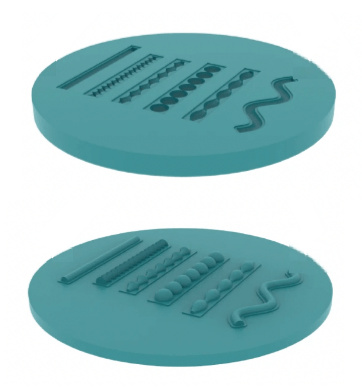Microfabricated substrates: cylinder, catenoids, pseudospheres, spheres, unduloid and sinusoidal cylinder. Length of each element approximately 2mm. Each one was printed in a concave (top) and a convex version: the Gaussian curvature is the same, but the mean curvature is reversed. Images from Callens, S.J.P., Fan, D., van Hengel, I.A.J. et al. Emergent collective organization of bone cells in complex curvature fields. Nat Commun 14, 855 (2023).

Those environments had a variety of curvatures. They included unduloids, surfaces where the mean curvature $\frac{1}{2}(\kappa_1 + \kappa_2)$ is constant. (Unduloids were first published by Charles-Eugène Delaunay in 1841). When the mean curvature is zero, unduloids form a cylinder. As the mean curvature increases, they morph into a line of spheres. The researchers also used strings of pseudospheres (surfaces where the Gaussian curvature, the product of the principal curvatures $\kappa_1\kappa_2$, is constant and negative) and shapes called catenoids. Finally, they threw in a cylinder bent into a sinusoidal curve, which unlike its straight, flat cousin has regions of positive and negative Gaussian curvature.A representative sample result. The day-5 assessment of growth using a stain that picks up a key structural component of cells. Here the substrate is the convex print of an unduloid. Note the low concentrations on the “bumps” where both principal curvatures are positive, and also on the flat surrounding areas where both are zero. Scale bar: 100$\mu$m. Image from Callens, S.J.P., Fan, D., van Hengel, I.A.J. et al. Emergent collective organization of bone cells in complex curvature fields. Nat Commun 14, 855 (2023).

Techacute.com picked up this research on March 29 (Gwendoline Guy, “Can Geometry Help Your Organs Regenerate?”). Guy interviewed Zadpoor, who reminds us that geometry belongs to everybody. For use in the lab, it’s “easy, safe and inexpensive.”

### Persistent homology and the early universe.

Cosmology has been called “the physics of the universe”, but it is not an experimental science. Cosmologists develop theories to explain the origin and evolution of the universe and use them to generate models to be compared with observation. The currently most generally accepted model, the “standard $\Lambda$ Cold Dark Matter” model, depends on a small number of parameters, like the age of the universe and the Hubble constant governing its expansion. That includes a parameter labeled $\sigma_8$, which measures the initial fluctuations of mass density in a certain volume of space (the “8” comes from the size of that volume in appropriate units). It turns out that there is general agreement on the values of the main cosmological parameters except for $\sigma_8$. This issue is addressed in “Wasserstein distance as a new tool for discriminating cosmologies through the topology of large-scale structure” published in the Monthly Notices of the Royal Astronomical Society April 18, 2023. The authors, the Ukrainian-Italian team of Maksym Tsizh, Vitalii Tymchyshyn and Franco Vazza, ran a series of simulations of the evolution of one small sector of the universe (from our point of view) for various values of $\sigma_8$, while keeping the other parameters fixed.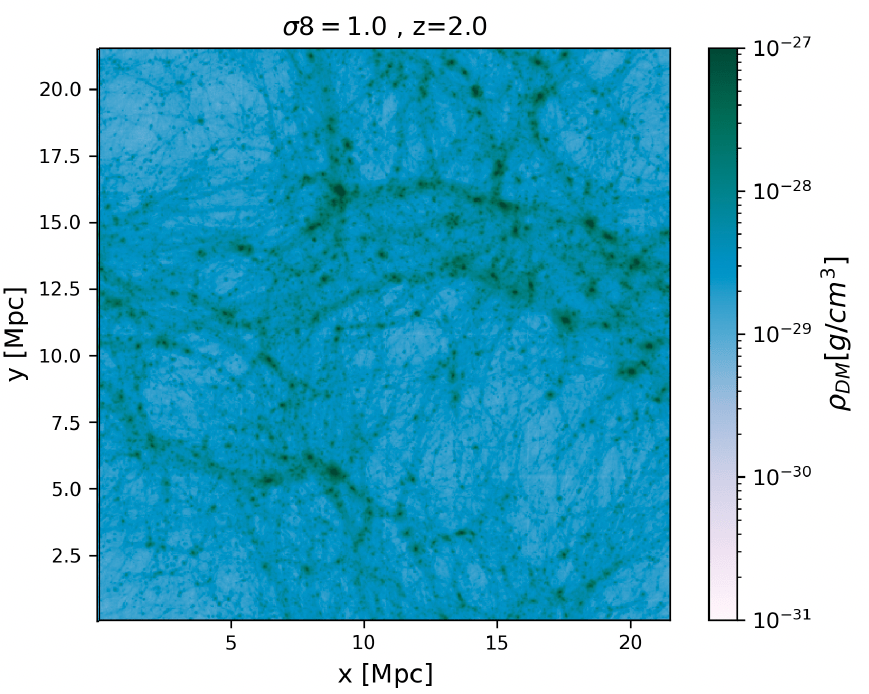The state at redshift $z=2$ (10.3 billion years ago) of two simulations of the evolution of the universe with the same initial conditions, one running with $sigma_8= 0.7$, the other with $sigma_8= 1.0$, cropped from the more complete set published in the article. These show $rho_{DM}$, the dark matter density, as projected onto our visual plane. One Mpc (Megaparsec) is 3.26 million light-years. Image courtesy of Maksym Tsizh and Franco Vazza.

For each value of $\sigma_8$, the simulations generate a picture like one of these for each of several different ages of the universe: each point represents a concentration of dark matter. For a given age, the authors want to measure the difference between the pictures corresponding to different values of $\sigma_8$. To do this, they use the Wasserstein distance; this is a way of measuring how different one cloud of points is from another. It involves “persistent homology”, a recently developed mathematical tool for taking a collection of data points and identifying clusters (and other, more complex structures) among them.

How persistent homology works. To get an intuitive idea of the procedure, imagine surrounding each point with a solid ball of radius $r$ centered at that point, and tracking how the union of all those solid balls changes shape as $r$ increases. The mathematical way of characterizing a shape starts by listing its Betti numbers. The zero-th Betti number $\beta_0$ counts the number of disjoint pieces. The first, $\beta_1$, counts the number of distinct holes in the shape. (Similarly, $\beta_k$ counts the number of its $k$-dimensional “holes.” A 2-dimensional hole, for instance, would be the hollow interior of a sphere.) Typically, as $r$ increases, disjoint pieces will agglomerate into larger units; at the same time, holes may form (“be born”) and may “die” when their centers get filled in, with analogous phenomena in higher dimensions.How persistent homology works. As the radius $r$ increases, a hole is born at $r=r_a$ and dies (is filled in) at $r_b$. Another hole is born at $r_c$ and dies at $r_d$. Meanwhile at $r=r_d$ the eleven initially disjoint pieces have merged into one.

One characteristic of the persistent homology of a space is the set of its barcodes. There is one for each dimension; it is a display of the [birth, death] intervals for each of the holes (see for example Figure 4 in this article). Finally, the Wasserstein distance between two distributions of points is computed, very roughly speaking, in terms of the differences between the ends of corresponding intervals in the barcodes of their persistent homology.

The goal of this work is to see if topological criteria like the Wasserstein distance could help pin down the value of the cosmological constant $\sigma_8$. By testing them on models generated with different values of $\sigma_8$, the authors can determine which criteria are the most sensitive, and could be most usefully applied to actual observational data. In their conclusions, the authors report that the Wasserstein distance gives the best $\sigma_8$ discrimination when used with images of the universe at earlier epochs, and that the distance calculated using dimension-0 barcodes shows more sensitivity to changes in $\sigma_8$ than calculations with higher-dimensional ones.

On a more terrestrial note, Franco Vazza started this collaboration with Maksim Tsizh just after the start of the current war in Ukraine. He remains active in encouraging Ukrainian astrophysicists to work with him and his department at the University of Bologna.